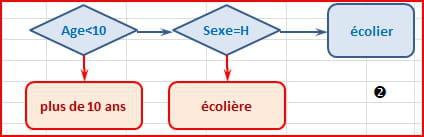The logic functionsA short explanation on the use of the following functions: IF, AND, OR, NOT
"If the note in A1 is greater than or equal to 19, then display the number 20, otherwise display A1" can be expressed as: =IF(A1>=19;20;A1)
"If the data in A1 is "Reset", then subtract 10%, otherwise keep A1", can be expressed as: =A1*IF(A1="Reset";1-10%;1)
"If the note in A1 is greater than 12, then display "continue"; if A1> 18, then add "that's fine" or display "satisfactory". But if A1 <= 12, then display "to be improved" , can be expressed as
=IF(A1>12;"continue, " & IF(A1>18;"that's fine";"satisfactory");"to be improved")
or the using the following formula:
=IF(A1<12;"to be improved";SI(A1<18;"continue, satisfactory";"continue, to be improved"))

If the note in A1 is less than 4, then display "nil"; if A1 is between 4 and 8, then display "not enough"; if A1 is between 8 and 12, then display "mid"; if A1 is between 12 and 16, then display "good", otherwise display "very good", can be expressed as:
=IF(A1<4;"nil";IF(A1<8;"not enough";IF(A1<12;"mid";SI(A1<16;"good";"very good"))))
Display "The Countess" if all these conditions are met: A2(sex)=female, B2(status)=married, C2(husband)=count and D2(score)=present, otherwise display "Hello" can be expressed as:
=IF(AND(A2="woman";B2="married";C2="count"; D2="present");"The Countess !";"Hello")
Display "Drive" if A3 contains the following data: plane, formula 1, moto, display "Driver" if A3 contains "car" or "work" otherwise display "?", can be expressed as:
=IF(OR(A3="plane";A3="formula 1";A3="moto");"Drive";IF(OR(A3="car";A3="work");"Driver";"?"))
=IF(INT(A3)=A3;"integer";"decimal") can also be written as
=IF(NOT(INT(A3)=A3);"decimal";"integer")

=IF(A4<>"french";"foreigner";"european") can also be written as
=IF(NOT(A4="french");"foreigner";"european")
The AND, OR and NOT are commonly used in association with the IF function.